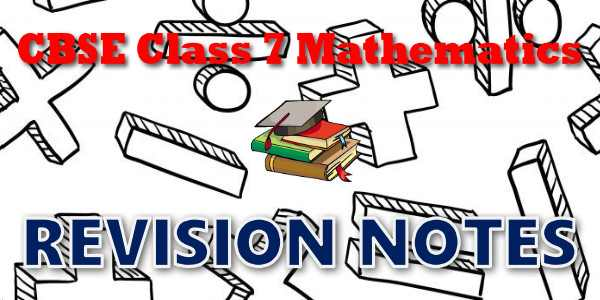No products in the cart.

# CBSE - Class 07 - Mathematics - CBSE Revision Notes## CBSE, JEE, NEET, CUET

Question Bank, Mock Tests, Exam Papers

NCERT Solutions, Sample Papers, Notes, Videos

## CBSE Revision Notes for Class 07 Mathematics

Integers
Integers## myCBSEguide App

Complete Guide for CBSE Students

NCERT Solutions, NCERT Exemplars, Revison Notes, Free Videos, CBSE Papers, MCQ Tests & more.

Fractions and Decimals
Fractions And Decimals
Data Handling
Data Handling
Simple Equations
Simple Equations
Lines and Angles
Lines And Angles
The Triangle and its Properties
The Triangle And Its Properties
Congruence of Triangles (Deleted)
Congruence Of Triangles
Comparing Quantities
Comparing Quantities
Rational Numbers
Rational Numbers
Practical Geometry (Deleted)
Practical Geometry
Perimeter and Area
Perimeter And Areas
Algebraic Expressions
Algebraic Expressions
Exponents and Powers
Exponents And Powers
Symmetry
Symmetry
Visualising Solid Shapes
Visualising Solid Shapes### Student Subscription

##### Unlock the exclusive content designed for the toppers## myCBSEguide App

Complete Guide for CBSE Students

NCERT Solutions, NCERT Exemplars, Revison Notes, Free Videos, CBSE Papers, MCQ Tests & more.

# CBSE Revision Notes for class 7 Mathematics## CBSE Class 7 Notes and Key Points

• CBSE Revision notes for Class 7 Mathematics PDF
• CBSE Revision notes Class 7 Mathematics – CBSE
• CBSE Revisions notes and Key Points Class 7 Mathematics
• Summary of the NCERT books all chapters in Mathematics class 7
• Short notes for CBSE class 7th Mathematics
• Key notes and chapter summary of Mathematics class 7
• Quick revision notes for CBSE exams

## CBSE Class 7 Mathematics Chapter-wise Revision Notes

• Chapter 1 – Integers
• Chapter 2 – Fractions and Decimals
• Chapter 3 – Data Handling
• Chapter 4 – Simple Equations
• Chapter 5 – Lines and Angles
• Chapter 6 – The Triangle and its Properties
• Chapter 7 – Congruence of Triangles
• Chapter 8 – Comparing Quantities
• Chapter 9 – Rational Numbers
• Chapter 10 – Practical Geometry
• Chapter 11 – Perimeter and Area
• Chapter 12 – Algebraic Expressions
• Chapter 13 – Exponents and Powers
• Chapter 14 – Symmetry
• Chapter 15 – Visualising Solid Shapes

Key Notes for CBSE Board Students for Class 7. Important topics of all subjects are given in these CBSE notes. These notes will provide you overview of the chapter and important points to remember. These are very useful summary notes with neatly explained examples for best revision of the book.

## CBSE Class-07 Revision Notes and Key Points

CBSE notes for class 7 Maths, Science and Social Science with option to download in PDF format. The notes includes all the concepts given in text book and covers the whole syllabus as prescribed by CBSE, New Delhi. CBSE key notes are free to download.

CBSE Class 6 Mathematics
Revision Notes
CHAPTER – 2
Whole Numbers

• The numbers 1, 2, 3, …… which we use for counting are known as natural numbers.
• If you add 1 to a natural number, we get its successor. If you subtract 1 from a natural number, you get its predecessor.
• Every natural number has a successor. Every natural number except 1 has a predecessor.
• If we add the number zero to the collection of natural numbers, we get the collection of whole numbers. Thus, the numbers 0, 1, 2, 3, ... form the collection of whole numbers.
• Every whole number has a successor. Every whole number except zero has a predecessor.
• All natural numbers are whole numbers, but all whole numbers are not natural numbers.
• We take a line, mark a point on it and label it 0. We then mark out points to the right of 0, at equal intervals. Label them as 1, 2, 3, .... Thus, we have a number line with the whole numbers represented on it. We can easily perform the number operations of addition, subtraction and multiplication on the number line.
• Addition corresponds to moving to the right on the number line, whereas subtraction corresponds to moving to the left. Multiplication corresponds to making jumps of equal distance starting from zero.
•  Adding two whole numbers always gives a whole number. Similarly, multiplying two whole numbers always gives a whole number. We say that whole numbers are closed under addition and also under multiplication. However, whole numbers are not closed under subtraction and under division.
• Division by zero is not defined.
• Zero is the identity for addition of whole numbers. The whole number 1 is the identity for multiplication of whole numbers.
• You can add two whole numbers in any order. You can multiply two whole numbers in any order. We say that addition and multiplication are commutative for whole numbers.
• Addition and multiplication, both, are associative for whole numbers.
• Multiplication is distributive over addition for whole numbers.
• Commutativity, associativity and distributivity properties of whole numbers are useful in simplifying calculations and we use them without being aware of them.
• Patterns with numbers are not only interesting, but are useful especially for verbal calculations and help us to understand properties of numbers better.## myCBSEguide

Trusted by 1 Crore+ Students## Test Generator

Create papers online. It's FREE.## CUET Mock Tests

75,000+ questions to practice only on myCBSEguide app